Water lilies

Water lilies are growing on the pond and their number is doubled every day. The whole layer is covered in 12 days. How many days will it cover 8 layers?

Result

d =  3

Solution:Leave us a comment of example and its solution (i.e. if it is still somewhat unclear...):Be the first to comment!To solve this example are needed these knowledge from mathematics:

Do you have a linear equation or system of equations and looking for its solution? Or do you have quadratic equation?

Next similar examples:

1. Sum-log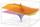The sum of two numbers is 32, the sum of their logarithms (base 10) is 2.2. Determine these numbers.
2. The city 3The city has 22,000 residents. How long it is expected to have 25,000 residents if the average annual population growth is 1.4%?
3. Theorem proveWe want to prove the sentence: If the natural number n is divisible by six, then n is divisible by three. From what assumption we started?
4. Unknown number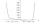I think number. If subtract from the twelfth square the ninth square I get a number 27 times greater than the intended number. What is this unknown number?
5. Ball gameRichard, Denis and Denise together scored 932 goals. Denis scored 4 goals over Denise but Denis scored 24 goals less than Richard. Determine the number of goals for each player.
6. Exponential equationSolve exponential equation (in real numbers): 98x-2=9
7. Logarithmic equation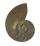Solve equation: log33(3x + 21) = 0
8. Insert into GPBetween numbers 5 and 640 insert as many numbers to form geometric progression so sum of the numbers you entered will be 630. How many numbers you must insert?
9. Powers 3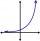2 to the power of n divided by 4 to the power of -3 equal 4. What is the vaule of n?
10. Intercept with axisF(x)=log(x+4)-2, what is the x intercept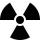After 548 hours decreases the activity of a radioactive substance to 1/9 of the initial value. What is the half-life of the substance?In the set R solve the equation: ?How many real roots has equation ? ?if ?, what is b?Solve for x: (4^x):0,5=2/64.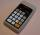If ?, then n is:Determine the first eight members of a geometric progression if a9=512, q=2